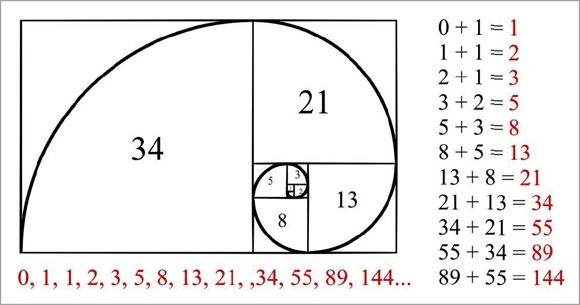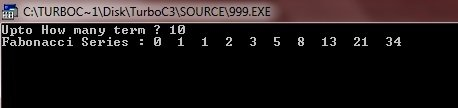# Print Fibonacci Series in C++

Watch out! This tutorial is over 3 years old. Please keep this in mind as some code snippets provided may no longer work or need modification to work on current systems.
Tutorial Difficulty Level

A “Fibonacci series” is a series of numbers in which each number ( Fibonacci number ) is the sum of the two preceding numbers. The simplest is the series 1, 1, 2, 3, 5, 8, etc. To print a Fibonacci series in C++, you have to ask the user to enter the total number of terms that he/she want to print fibonacci series upto the required number.Now to print our Fibonacci series; first print the starting two number of the Fibonacci series and make a while loop to start printing the next number of the Fibonacci series. Use the variable say… a, b and c. Place b in a and c in b then place a+b in c to print the value of c to make and print Fibonacci series as shown here in the following program.

When the above C++ program is compile and executed, it will produce the following result: